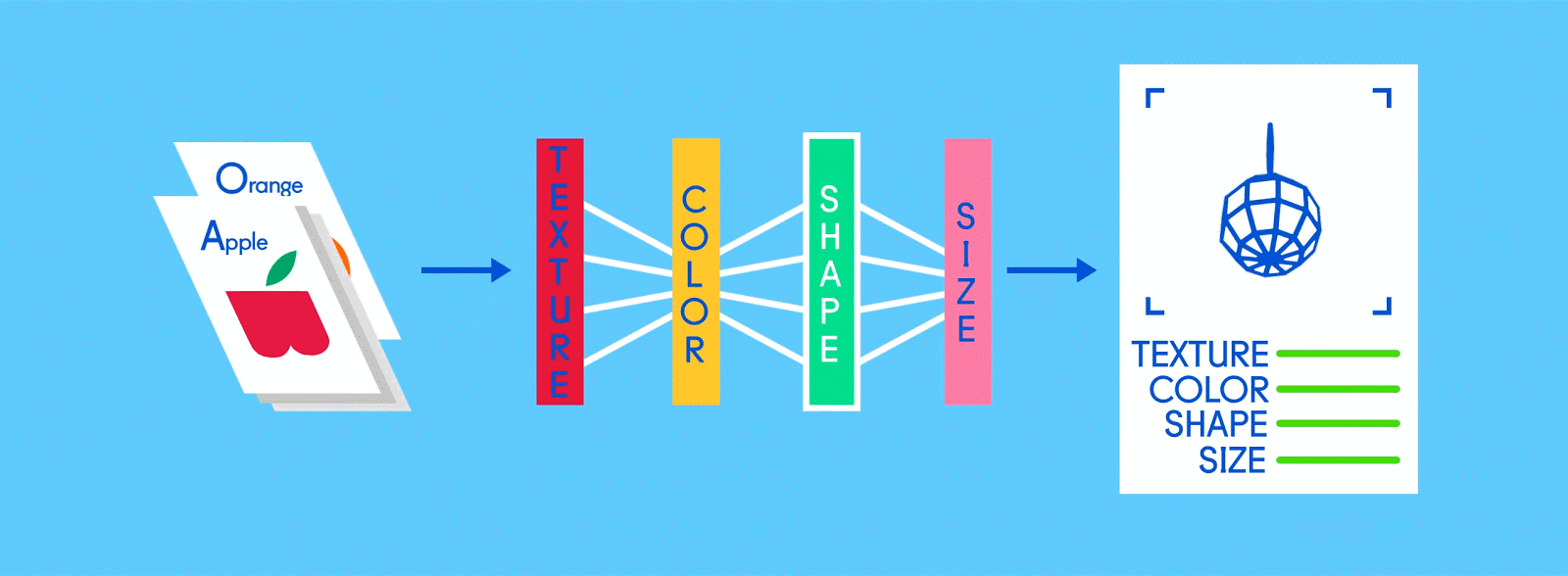# NEURAL NETWORK EASIEST WAY# LEARN NEURAL NETWORK EASIEST WAY.

Getting the easiest idea about the neural network is a tough thing for any beginners as it is a complicated concept to understand. First, we start our journey with an understanding of deep learning terminology.

WHAT IS DEEP LEARNING? (SHORT IDEA)

Deep learning is the process where machines try to learn many things in different forms such as images, audio, video, and much more. Its learn with training and then test on similar data and try to find the best suitable meaning from them. some neural networks are popular among the data science community, for example, CNN for image datasets, while LSTM for audio files.

WHY CALLED NEURAL NETWORK?

There are two different terminologies neural and network. it totally works like the human’s mind. Many neurons available in our minds and they are connected with a larger network. Each and every neuron has some value and all together are connected with each other.

NEURAL NETWORK IN DEEP LEARNING!!!

Concept of neural network tries to implement in machines so they can think and work like a human, for example, identify the picture(dog, cat,etc.). it can be achieved by a mathematical way first we should understand what is neurons? it is nothing but one number, in other words, all neurons have some value mostly it holds in between 0 and 1. The activation of neurons defines the structure of the network. If we take the example of grayscale images the value 0 means black and while holds the value 1. The brightness of neurons transfer from one layer to another and finally, the brightest neuron in the last layer called our output. In the video, you can identify the number 7.

WHY THE LAYERS ARE REQUIRED?!?

Neural networks required layers and it totally depends upon the problem and requirements. The solution of any problem define how many layers are requires and each layer has how many neurons? but now we are discussing what is the importance of layers? First of all, we should understand the work of the human mind. The human can identify objects in an easy manner. AS you can see every neuron have their own importance and all are interconnected with other layers. If we take an example of fruits identification of any machine so all layer identifies some special thing as you can see under some identify the color, another shape, and size; consequently, it generates the probability of any items and which have the highest probability it will machine assumptions.

PARAMETERS OF NEURAL NETWORK

Every neuron connected with each other that is why weights are required to connect each other. As you can see 7 digit example second layer 16 neurons are connected with first layer 784 neurons. Initially, we randomly assign weights, it might be a negative or positive number. But now we convert the weight in between 0 and 1 with the usage of activation functions. There are different types of activation functions and Sigmoid was popular among them which is convert the value between 0 and 1, whereas RELU is gaining popularity nowadays. Now, we understood the value of neurons which define as (a1,a2….an), weights of all the links in between neurons assign with w1,w2 ….own. Different activation functions use for NN. So we can assign neural network with σ(a1w1+a2w2+a3w3+……..anwn+ ”BIAS”)

WHAT IS BIAS AND ITS IMPORTANCE

It is an additional parameter in the Neural Network which is used to adjust the output along with the weighted sum of the inputs to the neuron. Thus, Bias is a constant which helps the model in a way that it can fit best for the given data. Two-layer neural network can be seen with the bias and without bias.

Due to the absence of bias, the model will train over point passing through origin only, which is not in accordance with a real-world scenario. Also with the introduction of bias, the model will become more flexible.

It helps the model to learn the patterns and it acts like an input node that always produces constant value 1 or other constant. Because of this property, they are not connected to the previous layer.

Bias units are not connected to any previous layer, it is just appended to the start/end of the input and each hidden layer, and is not affected by the values in the previous layer.

Now, we have to gain the knowledge of the training process of the neural network, how it updates the weight and bias values, and how it predicts value based on training. These kinds of questions will discuss in the next blog. So, keep connected and get knowledge as much as possible.